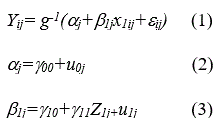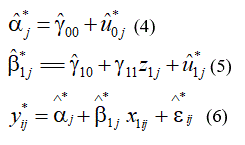Implementing Nonparametric Residual Bootstrap Multilevel Logit Model with Small Number of Level-2 UnitsResearch Article

Austin Biom and Biostat. 2017; 4(1): 1034.

# Implementing Nonparametric Residual Bootstrap Multilevel Logit Model with Small Number of Level-2 Units

Wang Y1*, Song L2 and Wang J1

1Departments of Pediatrics and Biostatistics, The George Washington University School of Medicine, USA

2Merck & Company, Inc., Merck Research Laboratory, Biostatistics and Research Decision Science, USA

*Corresponding author: Wang Y, Children's National Health System, Department of Pediatrics and Biostatistics, The George Washington University School of Medicine, 111 Michigan Avenue, NW, Washington, DC 20010, USA

Received: February 01, 2017; Accepted: March 16, 2017; Published: March 27, 2017

## Abstract

It is a challenge to analyze hierarchically structured data with either numerical or categorical response variables when number of groups (e.g., level 2 units) is small. Even sample size is large, a small number of groups would cause downward bias in standard errors of parameter estimates in multilevel modeling, thus the test statistics would be enlarged and the type I error would be inflated. Both parametric and nonparametric residual bootstrap approaches have been developed to deal with a small number of groups in multilevel modeling when the response variable is numeric. However, the corresponding approach is limited for multilevel modeling with categorical response variables, ex. binary outcome. To fill the gap, we have developed an approach by implementing nonparametric residual bootstrap multilevel logit model for binary data with small number of groups using SAS macro. With simulated data for modeling binary response variable with a small number of groups, our results showed explicit advantage of the nonparametric residual bootstrap approach over the approach using the default estimator -- Residual Pseudo-Likelihood (RSPL) –in SAS Proc Glimmix.

Keywords: SAS Proc Glimmix; SAS macro; Multilevel model; Residual Bootstrap Multilevel Logit Model; Bias

## Introduction

In statistics, there are two important concepts: consistence and bias of a parameter estimate. Consistence means that a parameter estimate  converges to its unknown true parameter θ when sample size n→∞, while bias of a parameter estimate  refers to the difference between  and θ. Bias can be fixed bias due to the system itself or random bias/ variability due to sampling errors. Evidences show that Maximum Likelihood Estimates (MLEs) of variance components are generally downwardly biased [1,2] with multilevel logit model using generalized linear mixed procedure in SAS. When the number of groups (level-2 units) is small, the bias of variance components was downward for correlated binary data [3,4]. Of many factors which can result in the downward estimation of variance components, the estimators and the assumption are the most important. First, MLE estimates of variance are smaller than Ordinary Least Square (OLS) estimates because the denominator in the formula of MLE uses the sample size n instead of n minus the rank of an independent variable matrix in OLS; Second, both level-1 and level-2 residuals are assumed to have normal distributions. However, to ensure such an assumption holds, the number of groups (number of level-2 units) have to be large. Unfortunately, the assumption of a normal distribution for level-2 residuals may not hold in real research because the number of groups is often small. The downward bias of variance components implies the smaller standard error, resulting in bigger test statistics and therefore, inflating type I error in a hypothesis test. In addition, the downward bias of variance components will result in a shorter confidence interval, thus the claimed coverage probability of 95% Confidence Interval (CI) will be less than stated [5,6]. The ways to correct the downward bias of standard errors include, but not limited to: asymptotic bias correction, Jackknife, and bootstrap. Restricted or Residual Maximum Likelihood (REML) approach  used in the SAS Proc Mixed model for numerical data, and Residual Pseudo-likelihood (RSPL) analog to REML used in SAS Proc Glimmix for categorical data, belong to the category with asymptotic bias correction. REML takes into account of fixed effects to maximize the likelihood function while RSPL is casted in terms of Taylor expansion to maximize the pseudo likelihood . Both REML and RSPL can reduce, to some extent, the bias in estimation of some complex variance. Firth  proposed another approach by adding a first order bias term of the maximum likelihood estimator to the score function to prevent the bias from occurring. In Jackknife procedure , the leave-one-out estimators are used for bias correction with order asymptotic bias smaller than O(n-1) . Bootstrap is a collection of methods following the bootstrap framework to improve the accuracy of inference. Three kinds of bootstrap methods are available: (1) case resampling through repeated sampling from original data with replacement, (2) parametric residual bootstrap with residual resample’s randomly drawn from normal distributions with replacement, (3) nonparametric residual bootstrap with residuals randomly drawn from new transformed residuals with replacement. Although both of the parametric residual bootstrap and nonparametric residual bootstrap are often used, the non-parametric residual bootstrap is more preferred because it provides more accurate inferences through correction of standard error or variance term  than parametric residual bootstrap. Wang et al.  developed an approach to conduct the nonparametric residual bootstrap multilevel modeling to deal with small number of groups for continuous response variables using a SAS macro. However, the corresponding modeling approaches are not available for categorical (e.g., binary) response variables. To the best of our knowledge, the present study is by far the first time to apply nonparametric residual bootstrap technique to modeling binary response variables in multilevel data with a small number of groups.

We gave a brief description of how to conduct on-parametric residual bootstrap multilevel modeling; and demonstrated how to implement nonparametric residual bootstrap multilevel logit model using our SAS macro, and then analyzed simulated data using residual bootstrap approach, as well as SAS Proc Glimmix with the default estimator RSPL. The results of the different modeling approaches were compared and findings were discussed.

## Methods

Non-parametric residual bootstrap multilevel logit model

The following hypothesized parsimonious multilevel logit model was used for demonstration of modeling hierarchically structured data with a binary response variable:where i=1, 2, …, i represents the ith level-1 unit, j=1, 2, …, j represents the jth group (level-2 units), g-1 is the inverse of a link function: a logit function for the logistic regression model. The level-2 residuals u0j and u1jhave bivariate normal distributions with zero means and unknown variances and covariance; and u0j and u1j are independent of the level-1 residual e1j which is assumed to have a normal distribution with the mean of zero and unknown variance.

When the number of groups is small, non-parametric residual bootstrap approach is applied for multilevel modeling (RBMLM) . The primary procedure of RBMLM is to transform both level-1 and level-2 residuals of a multilevel model, and then draw random samples of the transformed residuals with replacement to generate a large set of bootstrap samples. With a continuous response variable, the model has an identity link function. A new response variable is generated as the combination of the predicted value  and the transformed residual for each subject in each bootstrap sample. For multilevel logit model, the level-1 and level-2 residuals can be estimated from SAS Proc Glimmix procedure, and then transformed using the same approach described in Wang et al. . A new response variable in the bootstrap sample is generated by summing up the predicted log odds (i.e., logit) and the transformedlevel-1 residual. However, the generated new response variable is continuous in scale; we, therefore, need to transform it to a binary measure (0 vs 1) for final modeling. The specific steps for conducting non-parametric residual bootstrap multilevel logit model are described below.

Step 1: Run a multilevel logit model with the simulated dataset, save the level-1 and level-2 residuals, and then rescale the residuals by centering to ensure they have zero means. Next, transform the rescaled residuals into new residuals (see Appendix 1 in Wang, Carpenter, & Kepler ).

Step 2: Draw a random sample with replacement from the transformed level-1 and level-2residuals, separately.

Step 3: Use the transformed Level-2 residuals to estimate adjusted fixed coefficients.

Step 4: Use the adjusted fixed coefficients to estimate the predicted log odds (i.e., logit), and then generate a new response variable by summing up the estimated log odds and the transformed level-1 residual.

Let’s use the model shown in Eqs 1-3 to further describe Steps 3 and 4. After level-2 residuals are transformed, they are used to generate the adjusted fixed coefficients using Eqs. (4) and (5); and then generate a new continuous response variable using Eq. (6).Then Step 5 follows, in which a new binary response variableis generated by turning the generated numeric response variableinto a probability using the inverse of logit function, and then comparing  with a random number rij that was drawn from the uniform distribution of (0,1) for each individual. If prij>rij, then letelse Then, refit the model shown in Eqs 1-3, using the new binary variableas the response variable and save the model parameter estimates.

Step 6: Repeat Steps 2-6 for a total of (B-1) times (B=500 bootstrap samples in the present study) and append the B sets of model parameter estimates. The mean and standard deviation of the empirical distribution of the bootstrap estimates for particular parameter would be the bootstrap parameter estimate and its standard error, respectively.

On the basis of the SAS macro RBMLM developed by Wang et al. , we developed a SAS macro for nonparametric residual bootstrap multilevel logit model. Since Proc Glimmix used to analyze data with a generalized linear mixed model can provide residuals similar to Proc Mixed, it can be used to obtain the residual terms for logit models which cannot be obtained using traditional logistic regression. A multilevel dataset with 2500 observations consisting 50 groups (level-2 units) with 50 cases in each group was simulated for demonstrating the parsimonious multilevel logit model shown in Eqs. 1-3.

Simulation

First, the model was estimated using SAS Proc Glimmix procedure with the default estimator of Residual Pseudo-Likelihood (RSPL). Then, three sub-datasets were randomly selected from the simulated dataset with different number of groups (20, 15, and 10), in which the group size remained unchanged (i.e., 50 cases per group). Then both Proc Glimmix with the default estimator RSPL and our SAS macro were applied to model each of the three sub-datasets, respectively; and the parameter estimates were compared to “true” parameters. Assuming the dataset was simulated for a “target populations,” the regression coefficients (e.g., fixed-effect of x1, cross-level interaction effect of x1*z1) estimated from the dataset using RSPL are considered “true” parameters. If a regression coefficient estimated using a sub-dataset sampled from the original dataset deviated from the corresponding “true” coefficient, the deviation would be considered bias. The extent of bias was evaluated by coverage probability of the 95% C.I.